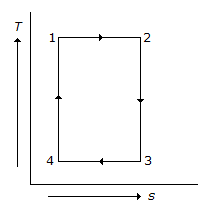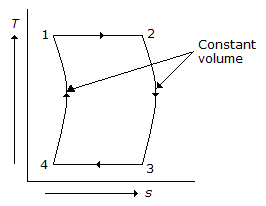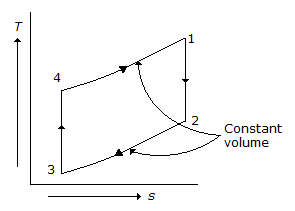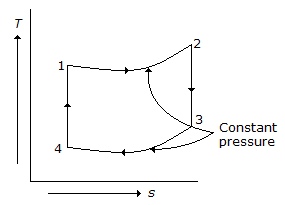# Mechanical Engineering - Thermodynamics

### Exercise :: Thermodynamics - Section 1

6.

An adiabatic process is one in which

 A. no heat enters or leaves the gas B. the temperature of the gas changes C. the change in internal energy is equal to the mechanical workdone D. all of the above

Explanation:

No answer description available for this question. Let us discuss.

7.

Water gas is obtained by passing air and a large amount of steam over waste coal at about 650°C.

 A. Correct B. Incorrect

Explanation:

No answer description available for this question. Let us discuss.

8.

Which of the following represents Otto cycle on temperature - entropy (T - s) diagram?

 A.B.C.D.Explanation:

No answer description available for this question. Let us discuss.

9.

When the gas is heated at constant volume, the heat supplied increases the internal energy of the gas.

 A. True B. False

Explanation:

No answer description available for this question. Let us discuss.

10.

Which of the following is the lightest and most volatile liquid fuel?

 A. Gasoline B. Kerosene C. Fuel oil